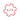# Shortest Non-repeating Substring

Given an alphanumeric string, find the shortest substring that occurs exactly once as a (contiguous) substring in it. Overlapping occurrences are counted as distinct. If there are several candidates of the same length, you must output all of them in the order of occurrence. The space is NOT considered as a valid non-repeating substring.

## Examples

Consider the following cases:

Case 1: If the given string is asdfsasa, the answer should be ['d', 'f']

Case 2: If the given string is sadanands,

the answer should be ['sa', 'ad', 'da', 'na', 'nd', 'ds']

Case 3: If the given string is wwwwwwww, the answer should be ['wwwwwwww']

## My Solution

Here is my solution in Python.

It is quite brute force. I am not sure about the order of find() and rfind() built-in methods in Python. Assuming these are $O(n)$, my algorithm is in $O(n^3)$. Please put your answers in comments below, if your answer has a better scaling.

The function definition that I use for finding non-empty non-repeating strings is recursive.

def findNsubString(s, n):
subS = []
for index in range(len(s)+1):
x = s[index:index+n]
if s.find(x) == s.rfind(x):
subS.append(x)
if subS:
return subS
else:
return findNsubString(s, n+1)

I call this method as follows to get the desired results:

#! /usr/bin/python
import argparse
# Parse Command Line Arguments
parser = argparse.ArgumentParser()
parser.add_argument("-s", "--string", default = "asda", help="Input")
args = parser.parse_args()
s = args.string
# Call Method to find smallest non-repeating sub-string
ans = findNsubString(s, 1)
print(ans)

A similar solution can also be written in JAVA or C++. The corresponding find() and rfind() methods in JAVA are called indexOf() and lastIndexOf(), respectively. In C++, these methods are called same as in Python.

Please feel free to put your method definition in any programming language.

Share This:

## Comments

### Stay in touch

Like the posts you see here? Sign up to get notified about new ones.

# Get The Best Of Posts Delivered To Your Inbox

Subscribe to my newsletter and stay updated.